Flow Efficiency helps you measure the actual work time against the Lead time. So, the efficiency can be measured by dividing Work Time by the combination of Work Time and Wait Time, and the higher the percentage, the better and smoother the process having less waste in the system and more agility to turn an idea or request into a workable feature.

#### Applying Filters

You can apply filters based on card attributes and the value stream stages to refine your data for the analytics. Moreover,  the time range for the analytics can also be adjusted using the Temporal Range Filter. Read more about them here.

## Flow Efficiency Scatterplot

This is a Scatter plot of card flow efficiency. Flow efficiency is defined as (work time / total time). Work time is defined as total time spent in In Progress columns. We subtract the Block time from effective work time as the most common reason for blocking among our customers is to indicate resource unavailability. Thus, the formula used here is the effective work time / total time. Flow efficiency of a card becomes available when a card exits.

Different shapes indicate the type of exit:

• Circle: move forward or is archived
• Triangle: move back
• Square: move to other value-stream
• Diamond: cards that were deleted or discarded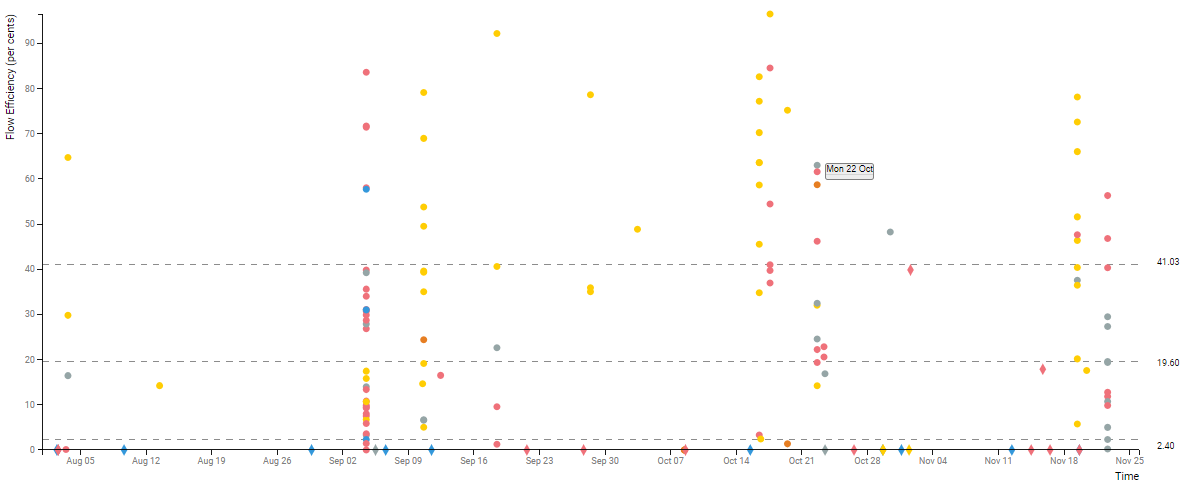You can further analyze the Scatterplot data based on the Quartile or the Percentile value and also view the Rolling average line for a user-driven number of days. To view such data, click the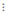icon from the widget menu, and then select any of the following options: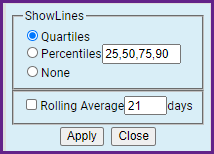#### Quartile

On the right of the scatter plot, we have a box-and-whisker plot as defined by John W. Tukey. Box-plot provides a non-parametric characterization of data without making any assumptions about the underlying distribution. The bottom and top of the box are the first and third quartiles, and the line inside the box is the second quartile (the median). The lower whiskers are drawn to the lowest datum still within the 1.5 IRQ ( interquartile range: q3 – q1) of the lower quartile, and the upper whisker is the highest datum still within the 1.5 IRQ of the upper quartile.

Box-and-whisker plot is often used to identify outliers in place of 3 sigmas when the underlying distribution is unknown. For comparison, for normal distribution, the 6 sigma range represents 99.73% of the total area, whereas the box-and-whisker range represents 99.3% of the area. The actual area being covered, however, depends on the nature of the distribution.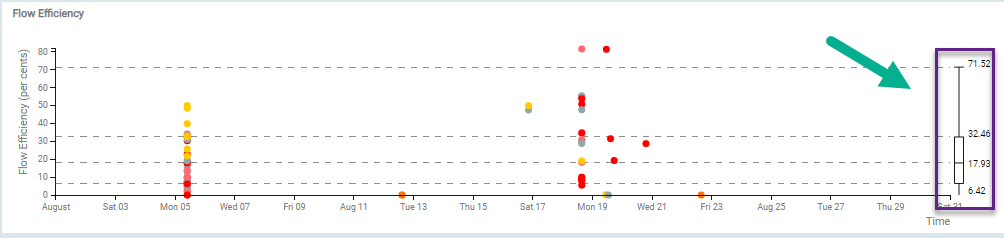#### Percentile

you enter the percentile values as per your requirement with the comma-separated format, the horizontal dotted lines demarcate the area of observation. Each area between the dotted lines represents the percentile value below which a percentage of average flow efficiency data falls.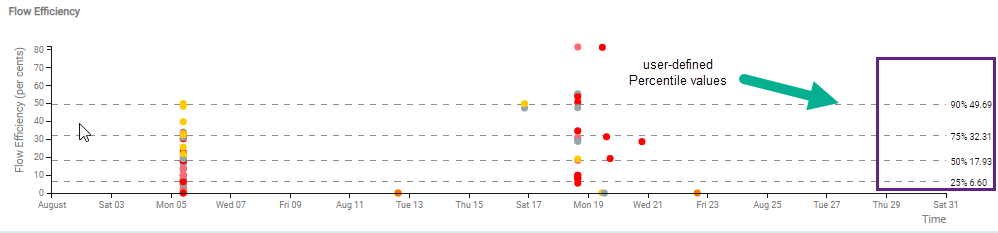#### Rolling Average

You can plot a line based on the rolling average of the flow efficiency for the n number of days. The rolling average is the moving average of the cards that are being worked upon in between the selected region of the value stream during the selected time interval. The line is formed by joining the series of data points of the average flow efficiency for a given time interval. It not only helps you understand the trend of the work time vs. wait time but also visualize the emerging pattern and detect any intermittent peak.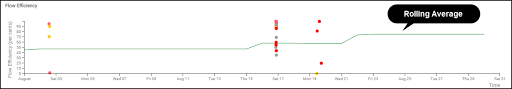## Flow Efficiency Histogram

A histogram of flow efficiency for cards that exit the selected value-stream region in the time interval selected in the Time Range Filter and pass other selected filtering criteria. The x-axis represents the flow efficiency in buckets of 10% and y-axis represents the count of cards whose flow efficiency falls in each bucket.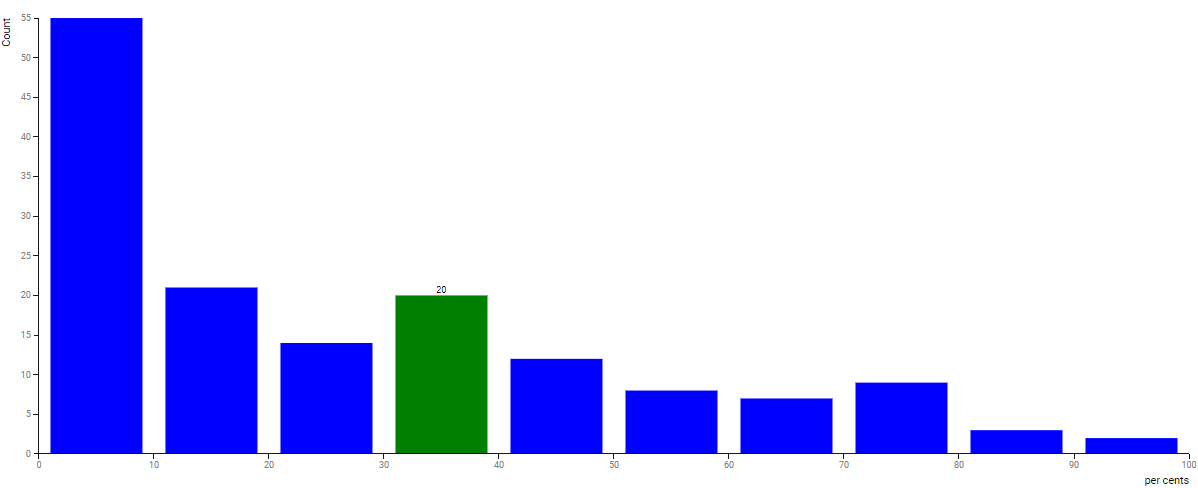## Work Time vs. Wait Time

This correlation graph shows the relation between effective work time and wait time for the cards that have completed their journey through the selected region of the value stream. Effective work time is defined as time spent in In-Progress columns subtracting the block time while in these columns.
The block time is considered to be wait time and therefore is added to the wait time. The x-axis represents wait time, the y-axis represents work time. The flow efficiency is shown using radial lines originating from [0,0].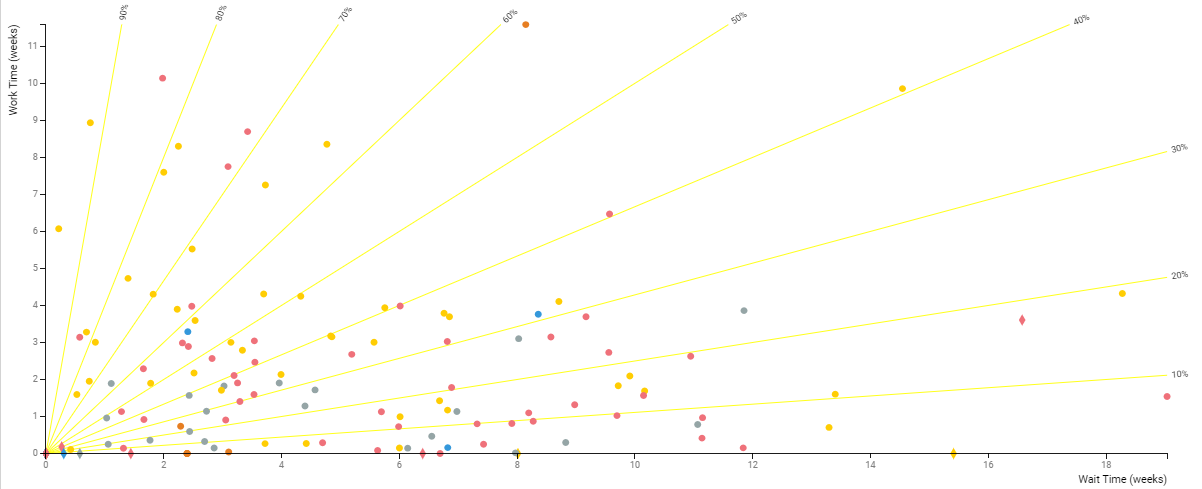Different shapes indicate the type of exit:

• Circle: move forward or is archived
• Triangle: move back
• Square: move to other value-stream
• Diamond: cards that were deleted or discarded